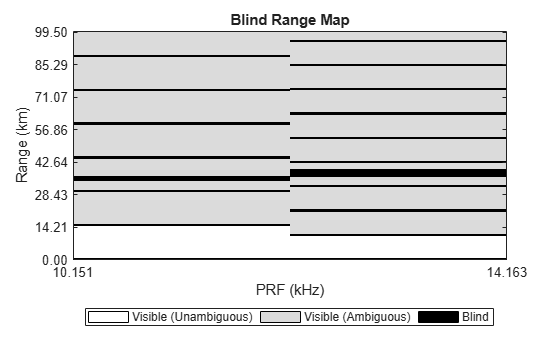# blindrangemap

Create blind range map for pulsed radar

Since R2023a

## Syntax

``BRM = blindrangemap(R,PRF,Rmin)``
``BRM = blindrangemap(R,PRF,Rmin,obstr)``
``BRM = blindrangemap(___,'RangeMask',rm)``
``[BRM,U] = blindrangemap(___)``
``blindrangemap(___)``

## Description

example

````BRM = blindrangemap(R,PRF,Rmin)` creates a blind range map for a monostatic pulse-Doppler radar for `BRM` for ranges `R`. The radar transmits at the pulse repetition frequency `PRF`. The minimum range of the radar is `Rmin`.```

example

````BRM = blindrangemap(R,PRF,Rmin,obstr)` also specifies obstructions in range `obstr`.```
````BRM = blindrangemap(___,'RangeMask',rm)` also specifies a range mask `rm`.```
````[BRM,U] = blindrangemap(___)` also returns the visibility proportion `U`.```
``blindrangemap(___)` plots the blind range map.`

## Examples

collapse all

Start with a radar transmitting $5\text{\hspace{0.17em}}\mu \mathrm{sec}$ long pulses. The radar covers the range from $0-100$ km. Create a blind range map when the pulse repetition frequencies are 5000, 10000, and 15000 kHz. Ranges from 18.5 km to 19.8 km and from 30 km to 32 km are obstructed due to clutter.

`R = linspace(0,100e3,1000);`

Choose the three PRFs at 5000, 10000, and 15000 kHz.

`PRF = [5e3,10e3,15e3];`

Compute the minimum range of the radar from the pulse duration.

```tau = 5e-6; rmin = time2range(tau,physconst('LightSpeed'));```

Add two clutter obstruction regions and create the blind range map.

```obs = [18.5e3 19.8e3;30e3 32e3]; blindrangemap(R,PRF,rmin,obs)```A pulse radar transmits at two pulse repetition frequencies (PRF): 10.151 kHz and 14.163 kHz. Set the minimum detectable range of the radar to 1000 m. Sidelobe clutter extends from 34.5 km to 36.5 km when transmitting at the first PRF, and from 36.3 km to 39.8 km when transmitting at the second PRF. Display the blind range map for ranges between 0 and 100 km at 1 km increments.

`R = linspace(0,100e3,1000);`

Set the minimum detectable range to 1000 m.

`Rmin = 1000;`

Set the two PRF values.

`PRF = [10151 14163];`

Account for blind ranges due to sidelobe clutter using the range mask input argument. First, initialize the map to indicate that all ranges are visible.

`rangeMask = false(numel(R),numel(PRF));`

Then, mask the sidelobe clutter at PRF = 10.151 kHz.

`rangeMask(R > 34500 & R < 36500,1) = true;`

Mask the sidelobe clutter at PRF =14.163 kHz.

`rangeMask(R > 36300 & R < 39800,2) = true;`

Display the blind range map.

`blindrangemap(R,PRF,Rmin,'RangeMask',rangeMask)`## Input Arguments

collapse all

Map range, specified as a length-J vector of positive values. The function computes the blind range map at these ranges. Units are in meters.

Example: `[4000,4500,6000]`

Data Types: `double`

Pulse repetition frequencies, specified as a length-N vector of positive values. Units are in Hz.

Example: `[10000,15000]`

Data Types: `double`

Minimum detection range, specified as a positive scalar or length-N vector of positive values. Ranges between 0 and `Rmin` are blind to the radar.

• If the radar transmits pulses of fixed duration, `Rmin` must be a scalar and the size of the blind zone is the same for all PRF values.

• If the radar transmits pulses with having a constant duty cycle, `Rmin` is a length-N vector and the size of the blind zone may be different for each PRF.

Blind zones also occur at integer multiples of the radar unambiguous range Ruamb = c/(2*PRF) where c is the signal propagation speed. Units are in meters.

Example: `40000`

Data Types: `double`

Obstructions in range, specified as a Q-by-2 matrix of positive values. The qth-row specifies a range obstruction in the form `[rstartq,rstopq]` where `rstartq` and `rstopq` are the start and stop ranges of the qth obstruction such that `rstopq``rstartq`. Targets located at the obstructed ranges or ranges ambiguous to the obstructed ranges are not visible to the radar. Such range obstructions are caused by, for example, clutter, multipath interference, or jammer interference. Entries in `BRM` that correspond to obstructed ranges are set to a logical one (true). Otherwise, they are zero (false). Units are in meters.

Example: `[30000;31000]`

Data Types: `double`

Range mask, specified as a J-by-N logical matrix. The rows of `rm` correspond to ranges in `R` and the columns correspond to pulse repetition frequencies in `PRF`. `rm``(j,n)` is a logical zero (false) if the `j`th range cell is visible to the radar at the `n`th frequency in PRF. `rm``(j,n)` is logical one (true) if the `j`th cell is blind to the radar at the `n`th PRF. `rm` can be used to specify ranges that are blind due to sidelobe clutter.

Data Types: `double`

## Output Arguments

collapse all

Blind range map, returned as a J-by-N logical matrix. Each row of `BRM` represents a range cell for the range equal to the jth entry in `R`. Each column of `BRM` corresponds to the nth pulse repetition frequency in `PRF`. If the jth range cell is visible to the radar at the nth `PRF`, `BRM``(j,n)` is a logical zero (false), otherwise `BRM``(j,n)` is a logical one (true).

Data Types: `logical`

Proportion of visible ranges, returned as a length-N vector of positive values where N is the number of pulse repetition frequencies in `PRF`. For each pulse repetition frequency, this argument indicates what proportion of ranges in `R` is visible.

Units are dimensionless.

## Version History

Introduced in R2023a